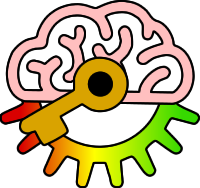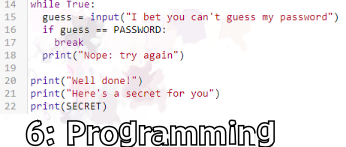# Edexcel GCSE Computer Science 2020

6.5.1: be able to write programs that use arithmetic operators (addition, subtraction, division, multiplication, modulus, integer division, exponentiation)

Keywords:Test your self on these keywords and definitions using the games below or
KeywordDefinition
-arithmetic operator which subtracts one number from another
-=arithmetic operator which decreases a variable by a given amount
*arithmetic operator which multiplies one number by another
**arithmetic operator which raises a number to a power
*=arithmetic operator which multiplies a variable by a given amount
/arithmetic operator which divides one number by another
//arithmetic operator which divides one number by another giving the result as an integer, always rounding down
/=arithmetic operator which divides a number by a given amount
%arithmetic operator which finds the modulus (remainder after integer division)
+arithmetic operator which adds two numbers together
+=arithmetic operator which increases a variable by a given amount
arithmetic operatorsymbol or characters used to perform a mathematical process on input data
Keyword games:
Resources: